Study Materials

# NCERT Solutions for Class 6th Mathematics

Page 7 of 7

## Chapter 3. Playing with Numbers

### Exercise 3.7

#### Exercise: 3.7

Q1. Renu purchases two bags of fertiliser of weights 75 kg and 69 kg. Find the maximum value of weight which can measure the weight of the fertiliser exact number of times.

Solution:

Renu purchases two bags of fertiliser of weights : 75 kg and 69 kg

Therefore, finding prime factorisation of 75 kg and 69 by dividing method:75 = 3 × 5 × 5

69 = 3 × 23

Therefore, HCF of (75, 69) = 3

Hence, Maximum Value of weight = 3 kg Answer

Q2. Three boys step off together from the same spot. Their steps measure 63 cm, 70 cm and 77 cm respectively. What is the minimum distance each should cover so that all can cover the distance in complete steps?

Solution: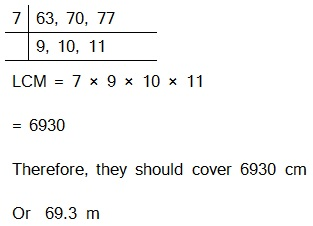Q3. The length, breadth and height of a room are 825 cm, 675 cm and 450 cm respectively. Find the longest tape which can measure the three dimensions of the room exactly.

Solution:

The measurement of longest tape = H.C.F. (825 cm, 675 cm, 450 cm)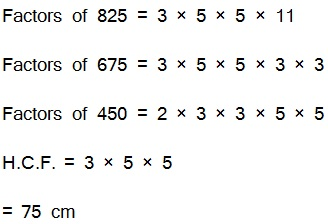Therefore, the longest tape is 75 cm.

Q4. Determine the smallest 3-digit number which is exactly divisible by 6, 8 and 12.

Solution: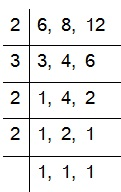L.C.M. of 6, 8 and 12

= 2 × 2 × 2 × 3

= 24

The smallest 3-digit number = 100

To find the number, we have to divide 100 by 24 1

00 = 24 × 4 + 4

Therefore, the required number = 100 + (24 – 4)

= 120.

Q 5. Determine the greatest 3-digit number exactly divisible by 8, 10 and 12.

Solution:

The greatest 3-digit number = 999

Now we find LCM of 8, 10 and 12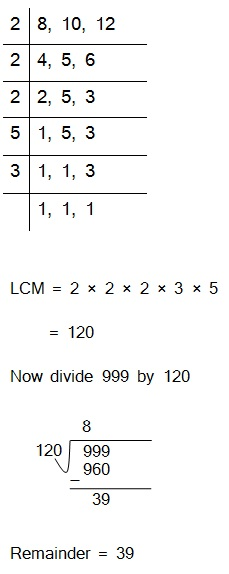So, When we subtract 39 from 999 we get 960.

Which is exactly divisible by 8, 10 and 12.

Hence, The greatest 3-digit number is 960.

Q6. The traffic lights at three different road crossings change after every 48 seconds, 72 seconds and 108 seconds respectively. If they change simultaneously at 7 a.m., at what time will they change simultaneously again?

Solution:

They meet together after LCM (48, 72, 108) seconds.

Now finding LCM,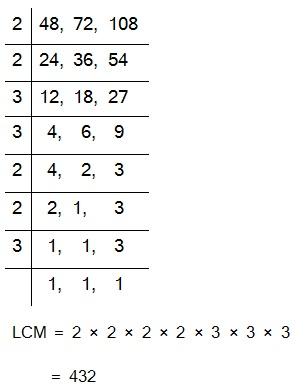Therefore, LCM (48, 72, 108) = 432

So these three lights burn together after 432 seconds.

432 seconds = 7 minutes 12 second

The time will be 7 a.m. +  7 minutes 12 second

= 7 : 07 : 12 a.m.

7. Three tankers contain 403 litres, 434 litres and 465 litres of diesel respectively. Find the maximum capacity of a container that can measure the diesel of the three containers exact number of times.

Solution:

The maximum capacity of that container = HCF (403, 434, 465)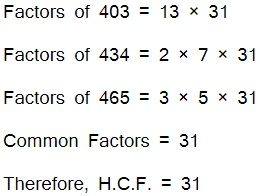Therefore, 31 litres of container is exact number which is required to measure the all containers' diesel.

8. Find the least number which when divided by 6, 15 and 18 leave remainder 5 in each case.

Solution:

The least number will be LCM(6, 15,18) + 5

Therefore, LCM(6, 15, 18)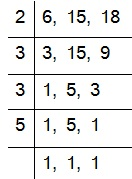LCM = 2 × 3 × 3 × 5

= 90

Now, 90 + 5 = 95

Hence required number 95 which leaves remainder as 5 on dividing by 6, 15, 18.

9. Find the smallest 4-digit number which is divisible by 18, 24 and 32.

Solution: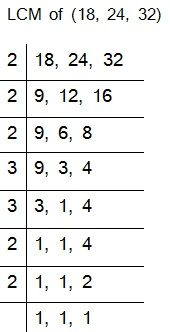LCM = 2 × 2 × 2 × 2 × 2 × 3 × 3

= 288

The smallest 4-digit number = 1000

Now, dividing 1000 by 288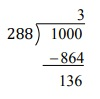Remainder is 136.

1000 needs more (288-136) = 152 to divide exactly by 288

Therefore, Smallest required number is 1000 + 152 = 1152

10. Find the LCM of the following numbers :

(a) 9 and 4

(b) 12 and 5

(c) 6 and 5

(d) 15 and 4

Solution:

(a) 9 and 4

9 and 4 has no common factors

Therefore, LCM = 9 × 4

= 36

Solution:

(b) 12 and 5

12 and 5 has no common factors

Therefore, LCM of 12 and 5 = 12 × 5

= 60

Solution:

(c) 6 and 5

6 and 5 has no common factors

Therefore, LCM of 6 and 5 = 6 × 5

= 30

Solution:

(d) 15 and 4

15 and 4 has no common factors

Therefore, LCM of 15 and 4 = 15 × 4

= 60

Observe a common property in the obtained LCMs. Is LCM the product of two numbers in each case?

Yes, the L.C.M. is equal to the product of two numbers in each case. And L.C.M. is also the multiple of 3 in each case.

11. Find the LCM of the following numbers in which one number is the factor of the other.

(a) 5, 20

(b) 6, 18

(c) 12, 48

(d) 9, 45

Solution:

(a) 5, 20

5 and 20 has common factor 5. Both are divisible by 5 and five is one of them.

Then, then greatest number will be LCM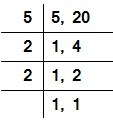LCM = 5 × 2 × 2

Therefore, LCM = 20

Solution:

(b) 6, 18

6 and 18 has common factor 6. and 6 is one of them.

Then the greatest number will be LCM.LCM = 2 × 3 × 3

Therefore, LCM = 18

Solution:

(c) 12, 48

12 and 48 has common factor 12 and 12 is one of them.

Then the greatest number will be LCM.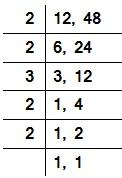LCM = 2 × 2 × 2 × 3

Therefore, LCM = 24

Solution:

(d) 9, 45

9 and 45 has common factor 9 and 45 is one of them.

Then the greatest number will be LCMLCM = 3 × 3 × 5

Therefore, LCM = 45

What do you observe in the results obtained?

We observe that both numbers belongs to same table and greatest number is the LCM.

Page 7 of 7

Chapter Contents: